# Electronic Warfare Basics: Pulse Repetition Interval (PRI)

In this first post of our series Electronic Warfare Basics we’ll cover an important foundational concept: Pulse Repetition Interval (PRI).A U.S. Navy EA-18G Growler — A key Navy Electronic Warfare Asset

# Introduction

A radar operates in a simple way:

1. Transmit an electromagnetic energy pulse at a frequency on the Electromagnetic Spectrum
2. “Listen” to the electromagnetic energy that is reflected back (if any) from the direction of transmission
3. Analyze the return signal (e.g. How long before I “heard” the return signal)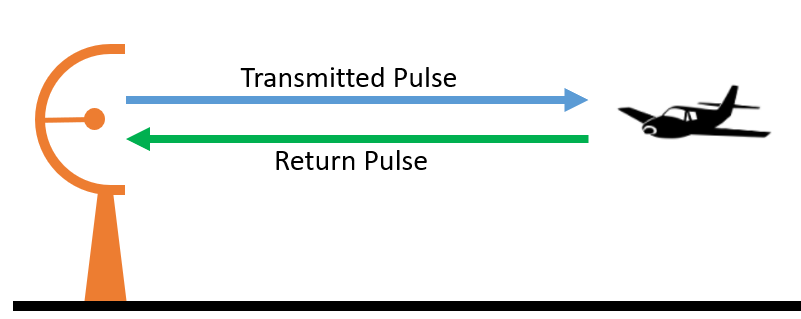Basic Radar Principles: Emit energy, wait for energy to return, and post process

A radar can only transmit electromagnetic energy in one direction, or beam position, at a time. This is a simple interpretation, but we’ll get into more detail later in the series. So, why only one direction? Because when a radar emits pulses in a single direction, it’s doing so to detect (possibly) an object in that direction, so it wants to keep listening in that direction until a return pulse is detected (or nothing — described later in the article).

# Calculation (Simple)

This basic understanding is all you need to begin your journey to understanding and analyzing PRIs. We don’t want to bore you with any fluff, so we’re going to get right into looking at an example PRI: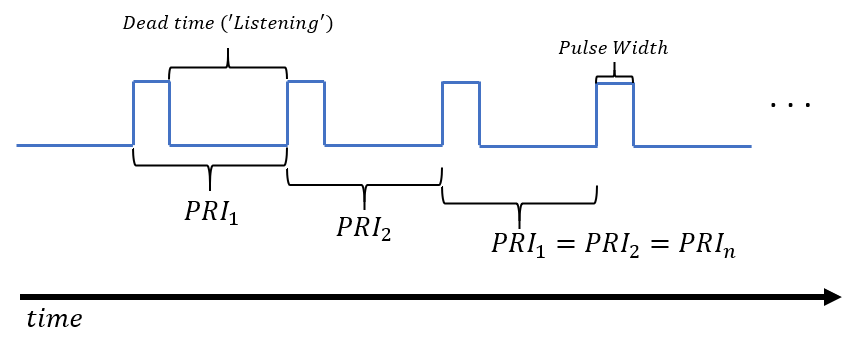Pulse Width (PW) is another emission property, and is the pulse duration.

In order to measure a PRI, the pulse needs to repeated (shown above). From there, you calculate a PRI as the time delta between the leading edge of one pulse to the leading edge of the next. Leading edge just implies the beginning of the energy emitted. So, PRI can be expressed by the following equation:

As an example, let’s evaluate the following:

It’s that simple . . . kind of. This is the most simple case, and in practice, modern radars can switch between a multitude of PRIs. But, it will always break down to optimizing this concept and subproblem shown above: What PRI(s) does a radar choose for its job?

# Example

Let’s examine the case of a very simple, 2D mechanically rotated early warning surveillance radar. Don’t worry, in later articles we’ll cover types of radars, scan patterns, beam steering, and all that fun stuff. For now, we only need to understand a few things:

• This radar rotates 360° in azimuth; think something like this.
• It’s fixed in elevation; meaning it’s emitting pulses at a fixed elevation (i.e. not ‘looking’ up and down while rotating).
• It’s emitting a single pulse at each beam position.
• The radar must listen in-between pulses for a return signal (‘dead time’ depicted above). It can’t wait forever, though, and PRI is important in determining how long to wait for that return signal.

Electromagnetic energy travels at the speed of light, typically denoted as c. So, I’ll ask you this: How long does a pulse of energy need to travel 225km? Let’s solve that below.

A pulse of energy (*in a vacuum) will travel 225km in 0.75ms! Now, let’s continue on this idea and I’ll ask: How long does a pulse need to travel 225km and back?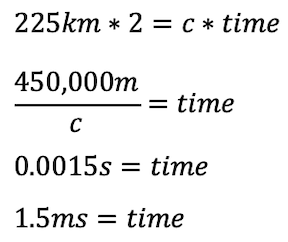In other words, 0.75ms * 2

What we just discovered is the equation for the Max Unambiguous Range of a radar!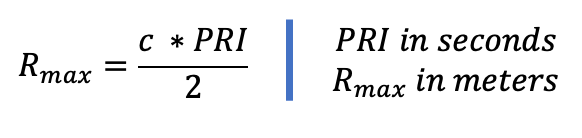Maximum Unambiguous Range Equation — A EW Fundamental concept. Simplified*

And, we used this equation to determine what our PRI should be if we want to continuously measure up to 225km! What do I mean up to 225km? Well, we know the time of the pulse n and how fast it travels (speed of light). So, if we ‘hear’ a return pulse after t seconds, we can use the equations above to determine our pulse reflected from an object x meters away. For example:

The reason our radar cannot go beyond our 225km range is because we would have ambiguity between return pulses. Our radar knows it requires 1.5ms for a pulse to return before emitting another pulse. Consider this, our radar pulse n reflects from an object at 250km, but we already emitted pulse n+1 by the time pulse n returns! Now we have ambiguity between did we see return pulse n+1 (detected an object within our 225km range) or did we see return pulse n? Now, don’t worry, there’s reasons this doesn’t become a large problem in practice, but this ambiguity problem is why a radar uses a PRI that fulfills it’s range requirement. We’ll get to this in another article.

# Wrapping it up

So, to recap, we learned what a PRI is and why it’s an important, fundamental characteristic in radar waveform design and analysis. There’s more to it, and we’ll continue to cover all the different PRI types and applications.

Note, most modern radars emit groups of pulses, and PRI simply implies the time delta between the leading edge of the first pulse of group n to the leading edge of the first pulse in group n+1. As such, it follows the same equations as above (Pulse Group Repetition Interval [PGRI] or Pulse Group Interval [PGI] are also used interchangeably in the context of PRI in modern nomenclature). The difference is that the time in PRI now includes the PW or duration of the Pulse Group. For completeness, the Maximum Unambiguous Range for a PG or pulse with large PW would be: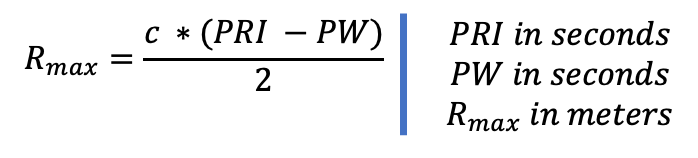Pulse Width (PW) is the duration of the pulse (equivalent to duration of Pulse Group)

We hope this article was helpful, and we’re planning on continuing the series! Thanks all!

--

--## Thomas Higginson

Software Engineer working on Cognitive EW capabilities, and human that enjoys making smiles. Welcome.